# 一、幾何變換的矩陣表示

## 1.1　平移的表示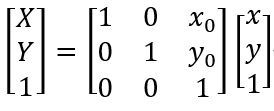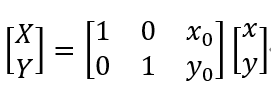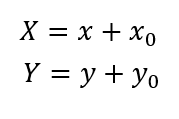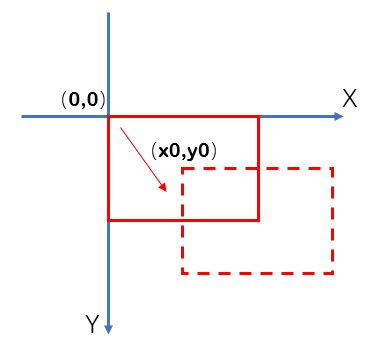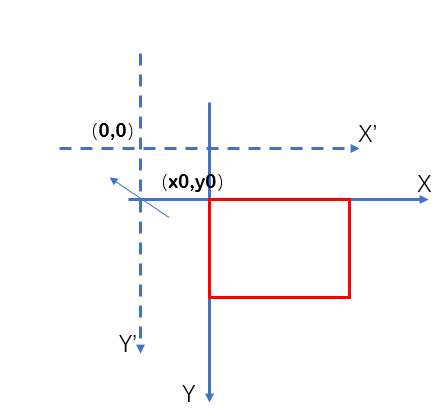## 1.2　以左上角為定點縮放的表示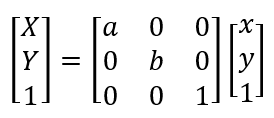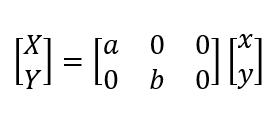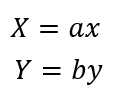## 1.3　以左上角點為中心旋轉的表示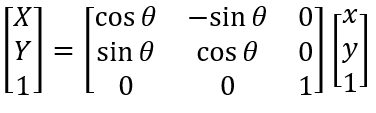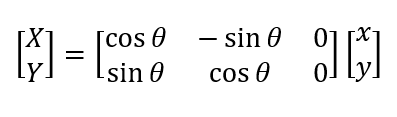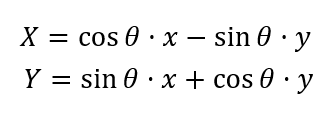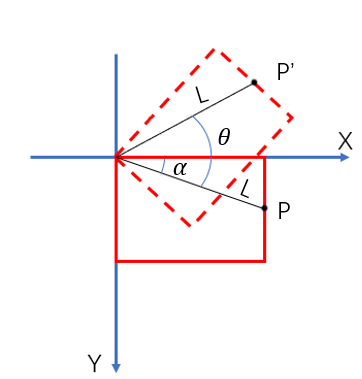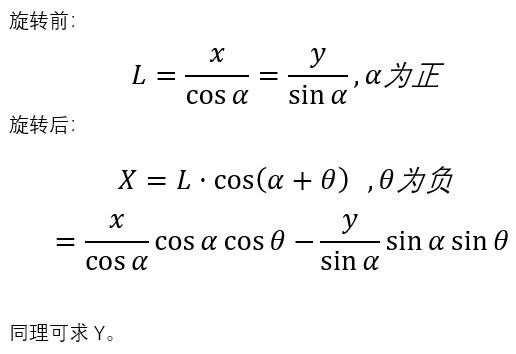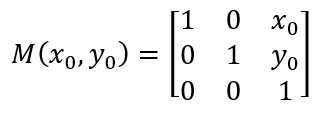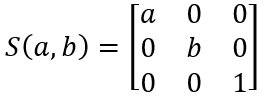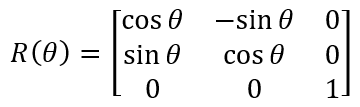## 1.4　以任一點為中心旋轉的表示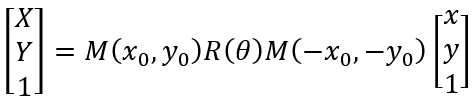## 1.5　以任一點為定點縮放的表示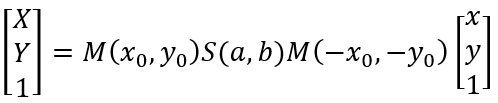# 二、旋轉傻烏龜OpenCV函數實現

OpenCV提供了仿射變換函數warpAffine。在輸入參數中，M表示變換矩陣，可以是平移、旋轉和縮放矩陣等；dsize是輸入圖像的大小；flags是插值方式，一般採用預設的雙線性插值。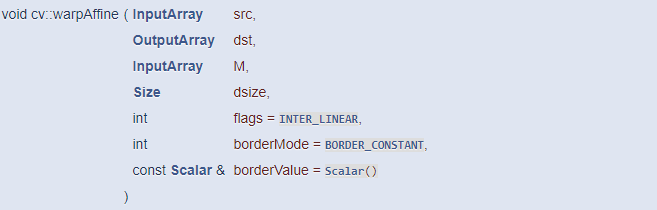``` 1 import cv2
2 import numpy as np
3 import time
4
6 size = img.shape[:-1]
7 cv2.namedWindow('img')
8
9 #平移矩陣
10 def GetMoveMatrix(x,y):
11     M = np.zeros((2, 3), dtype=np.float32)
12
13     M.itemset((0, 0), 1)
14     M.itemset((1, 1), 1)
15     M.itemset((0, 2), x)
16     M.itemset((1, 2), y)
17
18     return M
19
20 if __name__ == '__main__':
21
22     # shape和坐標是顛倒的
23     center_x = size/2
24     center_y = size/2
25     #計時
26     start_time = time.time()
27
28     for x in np.linspace(0,2*np.pi,100):
29         #角度、縮放
30         angle = -360*x/2/np.pi
31         scale = 0.2+0.2*np.sin(x)
32         #軌跡
33         path_x = x*50+100
34         path_y = (np.sin(x)+1)*100+100
35         #旋轉、平移矩陣
36         M1 = cv2.getRotationMatrix2D((center_x, center_y), angle, scale)
37         M2 = GetMoveMatrix(path_x-center_x,path_y-center_y)
38         #仿射變換
39         rotate = cv2.warpAffine(img,M1,size)
40         dst = cv2.warpAffine(rotate,M2,size)
41
42         # cv2.imshow('img',dst)
43         # cv2.waitKey(1)
44     #花費125ms
45     print(time.time()-start_time)```

# 三、旋轉傻烏龜自實現

這個自己用Python實現的話，性能就相當重要了，尤其是雙線性插值，如果不優化的話，慢得簡直可以讓你懷疑人生。比如，一般的是用兩個for迴圈迭代，代碼如下。在這個項目里，這個函數執行一次需要花費1.4s的時間。所以不優化的話，這隻烏龜真的是名副其實了！

``` 1 def InterLinearMap(img,size,mapx,mapy):
2
3     dst = np.zeros(img.shape,dtype=np.uint8)
4
5     for row in range(size):
6         for col in range(size):
7
8             intx = np.int32(mapx.item(row,col))
9             inty = np.int32(mapy.item(row,col))
10             partx = mapx.item(row,col)-intx
11             party = mapy.item(row,col)-inty
12             resx = 1-partx
13             resy = 1-party
14
15             if party==0 and partx==0:
16                 result=img[inty,intx]
17             else:
18                 result = ((img[inty,intx]*resx+img[inty,intx+1]*partx)*resy
19                           +(img[inty+1,intx]*resx+img[inty+1,intx+1]*partx)*party)
20
21             dst[row,col]=np.uint8(result+0.5)
22
23     return dst```

``` 1 #縮放矩陣
2 def GetResizeMatrix(scalex,scaley):
3     M = np.zeros((3,3),dtype=np.float32)
4
5     M.itemset((0,0),scalex)
6     M.itemset((1,1),scaley)
7     M.itemset((2,2),1)
8
9     return M
10 #平移矩陣
11 def GetMoveMatrix(x,y):
12     M = np.zeros((3, 3), dtype=np.float32)
13
14     M.itemset((0, 0), 1)
15     M.itemset((1, 1), 1)
16     M.itemset((2, 2), 1)
17     M.itemset((0, 2), x)
18     M.itemset((1, 2), y)
19
20     return M
21 #旋轉矩陣
22 def GetRotationMatrix(angle):
23     M = np.zeros((3, 3), dtype=np.float32)
24
25     M.itemset((0, 0), np.cos(angle))
26     M.itemset((0, 1), -np.sin(angle))
27     M.itemset((1, 0), np.sin(angle))
28     M.itemset((1, 1), np.cos(angle))
29     M.itemset((2, 2), 1)
30
31     return M```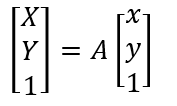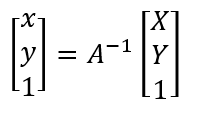``` 1 def WarpAffine(img,Mat,size):
2
3     rows = size
4     cols = size
5     #生成矩陣[X Y 1]
6     ones = np.ones((rows, cols), dtype=np.float32)
7     #gridx/gridy -> shape(rows,cols)
8     gridx,gridy= np.meshgrid(np.arange(0, cols),np.arange(0, rows))
9     #dst -> shape(3,rows,cols)
10     dst = np.stack((gridx, gridy, ones))
11
12     #求逆矩陣 M -> shape(3,3)
13     Mat = np.linalg.inv(Mat)
14     #獲得矩陣[x,y,1] -> shape(3,rows,cols)
15     src = np.tensordot(Mat,dst,axes=[[-1],])
16
17     #mapx/mapy -> shape(rows,cols)
18     mapx = src#坐標非整數
19     mapy = src#坐標非整數
20     #仿射出界的設為原點
21     flags = (mapy > rows - 2) + (mapy < 0) + (mapx > cols - 2) + (mapx < 0)
22     mapy[flags] = 0
23     mapx[flags] = 0
24     #雙線性插值
25
26     result = InterLinearMap(img, mapx, mapy)
27
28     return result```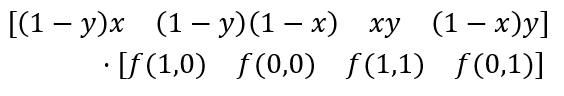代碼如下，經測試，執行一次該函數，花費時間為45ms，這要比原來的1.4s快多了（實在不知道該怎麼進一步優化了，mxy、img下表索引、求和各花了15ms）

```def InterLinearMap(img,mapx,mapy):

#(rows,cols)
inty = np.int32(mapy)
intx = np.int32(mapx)
nxty = 1+inty
nxtx = 1+intx
#(rows,cols)
party = mapy - inty
partx = mapx - intx
resy = 1-party
resx = 1-partx

#(4,rows,cols)
mxy = np.stack((resy*partx,resy*resx,partx*party, resx*party))
mxy = np.expand_dims(mxy,axis=-1)

#(4,rows,cols,3)
mf = np.stack((img[inty,nxtx],img[inty,intx],img[nxty,nxtx],img[nxty,intx]))

#res -> shape(rows,cols,3)
res = np.sum(mxy*mf,axis=0)
res = np.uint8(res+0.5)

return res```

```import cv2
import numpy as np

size = img.shape[:-1]
cv2.namedWindow('img')

#縮放矩陣
def GetResizeMatrix(scalex,scaley):
M = np.zeros((3,3),dtype=np.float32)

M.itemset((0,0),scalex)
M.itemset((1,1),scaley)
M.itemset((2,2),1)

return M
#平移矩陣
def GetMoveMatrix(x,y):
M = np.zeros((3, 3), dtype=np.float32)

M.itemset((0, 0), 1)
M.itemset((1, 1), 1)
M.itemset((2, 2), 1)
M.itemset((0, 2), x)
M.itemset((1, 2), y)

return M
#旋轉矩陣
def GetRotationMatrix(angle):
M = np.zeros((3, 3), dtype=np.float32)

M.itemset((0, 0), np.cos(angle))
M.itemset((0, 1), -np.sin(angle))
M.itemset((1, 0), np.sin(angle))
M.itemset((1, 1), np.cos(angle))
M.itemset((2, 2), 1)

return M

def InterLinearMap(img,mapx,mapy):

#(rows,cols)
inty = np.int32(mapy)
intx = np.int32(mapx)
nxty = 1+inty
nxtx = 1+intx
#(rows,cols)
party = mapy - inty
partx = mapx - intx
resy = 1-party
resx = 1-partx

#(4,rows,cols)
mxy = np.stack((resy*partx,resy*resx,partx*party, resx*party))
mxy = np.expand_dims(mxy,axis=-1)

#(4,rows,cols,3)
mf = np.stack((img[inty,nxtx],img[inty,intx],img[nxty,nxtx],img[nxty,intx]))

#res -> shape(rows,cols,3)
res = np.sum(mxy*mf,axis=0)
res = np.uint8(res+0.5)

return res

def WarpAffine(img,Mat,size):

rows = size
cols = size
#生成矩陣[X Y 1]
ones = np.ones((rows, cols), dtype=np.float32)
#gridx/gridy -> shape(rows,cols)
gridx,gridy= np.meshgrid(np.arange(0, cols),np.arange(0, rows))
#dst -> shape(3,rows,cols)
dst = np.stack((gridx, gridy, ones))

#求逆矩陣 M -> shape(3,3)
Mat = np.linalg.inv(Mat)
#獲得矩陣[x,y,1] -> shape(3,rows,cols)
src = np.tensordot(Mat,dst,axes=[[-1],])

#mapx/mapy -> shape(rows,cols)
mapx = src#坐標非整數
mapy = src#坐標非整數
#仿射出界的設為原點
flags = (mapy > rows - 2) + (mapy < 0) + (mapx > cols - 2) + (mapx < 0)
mapy[flags] = 0
mapx[flags] = 0
#雙線性插值

result = InterLinearMap(img, mapx, mapy)

return result

if __name__ == '__main__':

center_x = size/2
center_y = size/2

for x in np.linspace(0,2*np.pi,100):

angle = 360*x/2/np.pi
scale = 0.2+0.2*np.sin(x)

path_x = x*50+100
path_y = (np.sin(x)+1)*100+100

M = GetMoveMatrix(path_x,path_y)@GetRotationMatrix(x)\
@GetResizeMatrix(scale,scale)@GetMoveMatrix(-center_x,-center_y)

dst = WarpAffine(img,M,size)
cv2.imshow('img',dst)
cv2.waitKey(1)```• 本章內容參考官網即可,不做詳細說明,實踐出真知！ starUMl規則主要是在模型設計的約束條件 https://docs.staruml.io/user-guide/validation-rules 快捷鍵 https://docs.staruml.io/user-guide/keyboard-sh ...
• 這章比較簡單,主要是對視圖元素的樣式調整 主要是在視圖元素右下角設置,可以修改視圖元素的相關樣式 字體樣式 顏色 鏈接線樣式 對齊樣式 Stereotype Display-視圖元素的樣式屬性 菜單Format | Stereotype Display | [StereotypeDisplayKin ...
• Diagrams（圖）可以理解為畫布 1：創建圖 在右側的Model Explorer管理界面的第一個節點右鍵，或者選擇菜單中Model | Add Diagram | [DiagramType]都可以創建 2：Delete Diagram 選擇後滑鼠右鍵或菜單Edit中 3：Open Diagra ...
• 1：創建空的項目 創建項目可以按Ctrl+N或選擇菜單File| New，StarUML安裝打開後預設會有個空項目結構。 2：創建模板項目 可以通過選擇模板來啟動建模項目(會根據模板創建項目結構)。 要使用模板啟動項目，請從File| | New From TemplateName中選擇。 Star ...
• 為什麼用StarUML UML建模工具比較常見的PowerDesigner ROSE StarUML starUML-開源免費（1-2百M），PowerDesigner-精細和一體化（6-7百M），ROSE-大而全（3-4百M） 看個人設計目的，starUML（http://staruml.io/） ...
• 集合就像是一個購物車，可以將購買的所有商品的存放在一個統一的購物車中集合的概念現實生活： 很多的事物湊在一起數學中的集合： 具有共同屬性的事物的總體是一種工具類，是一種容器，裡面可以存儲任意數量的相同屬性的類。集合的作用在類的內部對數據進行組織簡單快速的搜索大數量的條目有的集合口，提供了一系列排列有... ...
• 通過getchar來取代cin不斷對緩衝區的操作，加快速度 調用 即可 ...
• 英文 | "Python Tips and Trick, You Haven't Already Seen" 原作 | Martin Heinz ( "https://martinheinz.dev" ) 譯者 | 豌豆花下貓 聲明 ：本文獲得原作者授權翻譯，轉載請保留原文出處，請勿用於商業或非法用 ...

• 本筆記摘抄自：https://www.cnblogs.com/PatrickLiu/p/8135083.html，記錄一下學習過程以備後續查用。 一、引言 今天我們要講行為型設計模式的第九個模式--訪問者模式。如果按老規矩，先從名稱上來看這個模式，我根本不能獲得任何對理解該模式有用的信息， 而且這個 ...
• 微信公眾號：【 "Dotnet9的博客" 】，網站：【 "Dotnet9" 】，問題或建議：【 "請網站留言" 】， 如果對您有所幫助：【 "歡迎贊賞" 】。 開源C WPF控制項庫系列： "（一）開源C WPF控制項庫《MaterialDesignInXAML》" "（二）開源C WPF控制項庫《Pan ...
• 如今，當談到 WPF 時，我們言必稱 MVVM、框架（如 Prism）等，似乎已經忘了不用這些的話該怎麼使用 WPF 了。當然，這裡說的不用框架和 MVVM，並不是說像使用 Winform 那樣使用 WPF，而是追本溯源，重識 WPF 與生俱來的綁定和命令的風采。 ...
• 本筆記摘抄自：https://www.cnblogs.com/PatrickLiu/p/8176974.html，記錄一下學習過程以備後續查用。 一、引言 今天我們要講行為型設計模式的第十個模式--備忘錄模式，先從名稱上來看。備忘錄模式可以理解為對某個對象的狀態進行保存，等到需要恢復的時 候，可以從 ...
• 前言 在兩年多以前就聽聞 Blazor 框架，是 .Net 之父的業餘實驗性項目，其目的是探索 .Net 與 WebAssembly 的相容性和應用前景。現在這個項目已經正式成為 Asp.Net Core 框架的一部分，公開了預覽版，官方教程也基本寫好上線了。就著這個機會，順便體驗一下這個框架用起來 ...
• .NET web開發者在開發過程中，一定都踩過的坑，明明修改了js文件，可是部署到生產環境，客戶反饋說：“還是報錯啊”。。然後一臉懵逼的去伺服器上看文件，確實已經更新了。有經驗的coder可能就想到了，肯定是客戶端瀏覽器緩存搞的鬼。 此時會告訴客戶，請Crtl+F5刷新一下，這時，客戶會說：“Ctr ...
• 哈嘍..大家好 很久沒有更新了，今天就來一篇最近開發用到的功能，那就是中英文切換，這個實際上也不是高大上，先說一下原理，在.NET Core框架中給我們提供了全球化的類，叫做Localization，其官方的文檔地址傳送門。 在我的項目中，我是這樣操作的，你想用別的方式，也可以看文檔自己去搞。這個已 ...
• WPF允許使用Image元素顯示點陣圖。然而，按這種方法顯示圖片的方法完全是單向的。應用程式使用現成的點陣圖，讀取問題，併在視窗中顯示點陣圖。就其本身而言，Image元素沒有提供創建和編輯點陣圖信息的方法。 這正是WriteableBitmap類的用武之地。該類繼承自BitmapSource，BitmapS ...